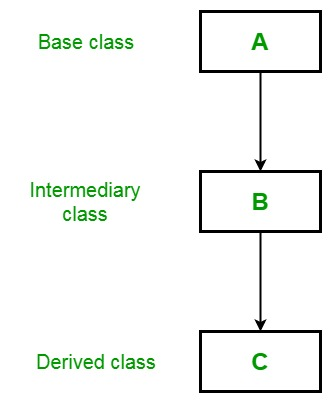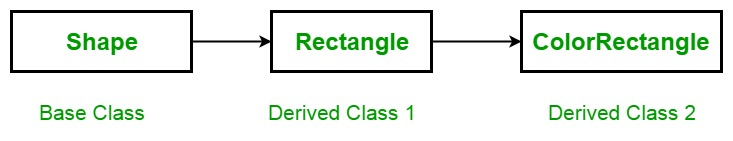GeeksforGeeks App
Open AppBrowser
Continue

## Related Articles

### Introduction:

In object-oriented programming, inheritance is the ability to create new classes that are derived from existing classes, known as base or parent classes. Inheritance allows the derived classes to inherit properties and methods of the base classes and to add new features or functionalities to the derived classes.

Inheritance is a powerful feature of object-oriented programming because it allows code reuse, increases code organization, and simplifies the development process. By using inheritance, developers can create new classes that inherit the common properties and behaviors of existing classes and then add new features or customize the inherited properties and behaviors.

There are several types of inheritance, including single inheritance, multiple inheritance, hierarchical inheritance, and multilevel inheritance. Each type of inheritance has its own advantages and disadvantages, and choosing the right type of inheritance depends on the specific needs of the application.

Overall, inheritance is a fundamental concept in object-oriented programming, and understanding its principles is essential for writing efficient and effective code

### Advantages of inheritance in object-oriented programming:

1. Code reuse: Inheritance allows developers to reuse code from existing classes, reducing the amount of new code that needs to be written. This makes the development process more efficient and faster.
2. Enhances code organization: Inheritance helps to organize code by creating a hierarchical structure of classes. This makes it easier to understand and manage the codebase.
3. Simplifies maintenance: By using inheritance, changes to the base class automatically apply to all the derived classes. This reduces the amount of time and effort required to maintain the codebase.
4. Promotes extensibility: Inheritance allows developers to extend the functionality of existing classes by adding new properties and methods to the derived classes.

### Disadvantages of inheritance in object-oriented programming:

1. Inheritance can lead to tight coupling between classes, making the code more difficult to modify and maintain.
2. Inheritance can lead to code duplication and redundancy, especially when multiple derived classes inherit from the same base class.
3. Inheritance can make the code more complex and harder to understand, especially when there are many levels of inheritance.
4. Inheritance can lead to the creation of large and complicated class hierarchies, which can be difficult to manage and may impact performance.
5. Overall, inheritance is a powerful tool in object-oriented programming, but it should be used judiciously, with careful consideration of the specific needs of the application..

In C#, multilevel inheritance refers to the ability to create a derived class that inherits from a base class, and then create another derived class that inherits from the first derived class. This creates a hierarchical structure of classes, where each class inherits the properties and methods of the classes above it in the hierarchy.

## C#

 `using` `System;` `public` `class` `Animal``{``    ``public` `void` `Eat()``    ``{``        ``Console.WriteLine(``"The animal is eating."``);``    ``}``}` `public` `class` `Mammal : Animal``{``    ``public` `void` `Walk()``    ``{``        ``Console.WriteLine(``"The mammal is walking."``);``    ``}``}` `public` `class` `Dog : Mammal``{``    ``public` `void` `Bark()``    ``{``        ``Console.WriteLine(``"The dog is barking."``);``    ``}``}` `public` `class` `Program``{``    ``public` `static` `void` `Main()``    ``{``        ``Dog myDog = ``new` `Dog();``        ``myDog.Eat();``        ``myDog.Walk();``        ``myDog.Bark();``    ``}``}`

Output

```The animal is eating.
The mammal is walking.
The dog is barking.```

In this example, the Animal class is the base class, the Mammal class is derived from Animal, and the Dog class is derived from Mammal. The Dog class inherits the Eat() method from the Animal class, the Walk() method from the Mammal class, and also has its own Bark() method.

When the Main() method is executed, it creates a new instance of the Dog class and calls its methods, including those inherited from the base and intermediate classes. The output of the program will be:

In the Multilevel inheritance, a derived class will inherit a base class and as well as the derived class also act as the base class to other class. For example, three classes called A, B, and C, as shown in the below image, where class C is derived from class B and class B, is derived from class A. In this situation, each derived class inherit all the characteristics of its base classes. So class C inherits all the features of class A and B.Example: Here, the derived class Rectangle is used as a base class to create the derived class called ColorRectangle. Due to inheritance the ColorRectangle inherit all the characteristics of Rectangle and Shape and add an extra field called rcolor, which contains the color of the rectangle.This example also covers the concept of constructors in a derived class. As we know that a subclass inherits all the members (fields, methods) from its superclass but constructors are not members, so they are not inherited by subclasses, but the constructor of the superclass can be invoked from the subclass. As shown in the below example, base refers to a constructor in the closest base class. The base in ColorRectangle calls the constructor in Rectangle and the base in Rectangle class the constructor in Shape.

## CSharp

 `// C# program to illustrate the``// concept of multilevel inheritance``using` `System;` `class` `Shape {` `    ``double` `a_width;``    ``double` `a_length;` `    ``// Default constructor``    ``public` `Shape()``    ``{``        ``Width = Length = 0.0;``    ``}` `    ``// Constructor for Shape``    ``public` `Shape(``double` `w, ``double` `l)``    ``{``        ``Width = w;``        ``Length = l;``    ``}` `    ``// Construct an object with``    ``// equal length and width``    ``public` `Shape(``double` `y)``    ``{``        ``Width = Length = y;``    ``}` `    ``// Properties for Length and Width``    ``public` `double` `Width``    ``{``        ``get` `{``               ``return` `a_width;``            ``}` `        ``set` `{``              ``a_width = value < 0 ? -value : value;``            ``}``    ``}` `    ``public` `double` `Length``    ``{``        ``get` `{``               ``return` `a_length;``            ``}` `        ``set` `{``              ``a_length = value < 0 ? -value : value;``            ``}``    ``}``    ``public` `void` `DisplayDim()``    ``{``        ``Console.WriteLine("Width and Length are "``                     ``+ Width + " and " + Length);``    ``}``}` `// A derived class of Shape``// for the rectangle.``class` `Rectangle : Shape {` `    ``string` `Style;` `    ``// A default constructor.``    ``// This invokes the default``    ``// constructor of Shape.``    ``public` `Rectangle()``    ``{``        ``Style = "``null``";``    ``}` `    ``// Constructor``    ``public` `Rectangle(``string` `s, ``double` `w, ``double` `l)``        ``: ``base``(w, l)``    ``{``        ``Style = s;``    ``}` `    ``// Construct an square.``    ``public` `Rectangle(``double` `y)``        ``: ``base``(y)``    ``{``        ``Style = "square";``    ``}` `    ``// Return area of rectangle.``    ``public` `double` `Area()``    ``{``        ``return` `Width * Length;``    ``}` `    ``// Display a rectangle's style.``    ``public` `void` `DisplayStyle()``    ``{``        ``Console.WriteLine("Rectangle ``is`  `" + Style);``    ``}``}` `// Inheriting Rectangle class``class` `ColorRectangle : Rectangle {` `    ``string` `rcolor;` `    ``// Constructor``    ``public` `ColorRectangle(``string` `c, ``string` `s,``                          ``double` `w, ``double` `l)``        ``: ``base``(s, w, l)``    ``{``        ``rcolor = c;``    ``}` `    ``// Display the color.``    ``public` `void` `DisplayColor()``    ``{``        ``Console.WriteLine("Color ``is` `" + rcolor);``    ``}``}` `// Driver Class``class` `GFG {` `    ``// Main Method``    ``static` `void` `Main()``    ``{``        ``ColorRectangle r1 = ``new` `ColorRectangle("pink",``                   ``"Fibonacci rectangle", 2.0, 3.236);` `        ``ColorRectangle r2 = ``new` `ColorRectangle("black",``                                   ``"Square", 4.0, 4.0);` `        ``Console.WriteLine("Details of r1: ");``        ``r1.DisplayStyle();``        ``r1.DisplayDim();``        ``r1.DisplayColor();` `        ``Console.WriteLine("Area ``is` `" + r1.Area());``        ``Console.WriteLine();` `        ``Console.WriteLine("Details of r2: ");``        ``r2.DisplayStyle();``        ``r2.DisplayDim();``        ``r2.DisplayColor();` `        ``Console.WriteLine("Area ``is` `" + r2.Area());``    ``}``}`

### Here are some recommended books for learning about inheritance in object-oriented programming:

1. “Head First Object-Oriented Analysis and Design” by Brett McLaughlin, Gary Pollice, and David West: This book is a great resource for beginners who want to learn about object-oriented programming concepts, including inheritance, in a fun and interactive way.
2. “Object-Oriented Programming in C++” by Robert Lafore: This book provides a comprehensive introduction to object-oriented programming in C++, including inheritance, polymorphism, and encapsulation.
3. “Java: A Beginner’s Guide” by Herbert Schildt: This book is a great resource for learning Java, including object-oriented programming concepts such as inheritance.
4. “Python for Everybody: Exploring Data in Python 3” by Charles Severance: This book is a great resource for learning Python, including object-oriented programming concepts such as inheritance.
5. “C# 8.0 and .NET Core 3.0 – Modern Cross-Platform Development” by Mark J. Price: This book is a comprehensive guide to learning C# and .NET Core 3.0, including object-oriented programming concepts such as inheritance.
6. These books are great resources for both beginners and experienced developers who want to learn more about inheritance in object-oriented programming.

My Personal Notes arrow_drop_up